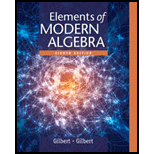# Exercise 37 − 39 can be generalized as follows: If 0 ≤ k ≤ n and the set A has n elements, then the number of elements of the power set P ( A ) containing exactly k elements is ( n k ) . Use this result to write an expression for the total number of elements in the power set P ( A ) . Use the binomial theorem as stated in Exercise 25 to evaluate the expression in part a and compare this result to exercise 27 and 37. (Hint: set a = b = 1 in the binomial theorem.) If n is a nonnegative integer and the set A has n elements, then the power set P ( A ) has 2 n If n ≥ 2 and the set A has n elements, then the number of elements of the power set P ( A ) containing exactly two elements is ( n 2 ) = n ( n − 1 ) 2 . If n ≥ 3 and the set A has n elements, then the number of elements of the power set P ( A ) containing exactly three elements is ( n 3 ) = n ( n − 1 ) ( n − 2 ) 3 ! . Let a and b be a real number, and let n be integers with 0 ≤ r ≤ n . The binomial theorem states that ( a + b ) n = ( n 0 ) a n + ( n 1 ) a n − 1 b + ( n 2 ) a n − 2 b 2 + ... + ( n r ) a n − r b r + ....... + ( n n − 2 ) a 2 b n − 2 + ( n n − 1 ) a b n − 1 + ( n n ) b n = ∑ r = 0 n ( n r ) a n − r b r Where the binomial coefficients ( n r ) are defined by ( n r ) = n ! ( n − r ) ! r ! , With r ! = r ( r − 1 ) ......... ( 2 ) ( 1 ) for r ≥ 1 and 0 ! = 1 . Prove that the binomial coefficients satisfy the equation ( n r − 1 ) + ( n r ) = ( n + 1 r ) for 1 ≤ r ≤ n### Elements Of Modern Algebra

8th Edition
Gilbert + 2 others
Publisher: Cengage Learning,
ISBN: 9781285463230### Elements Of Modern Algebra

8th Edition
Gilbert + 2 others
Publisher: Cengage Learning,
ISBN: 9781285463230

#### Solutions

Chapter
Section
Chapter 2.2, Problem 40E
Textbook Problem

## Expert Solution

### Want to see the full answer?

Check out a sample textbook solution.See solution

### Want to see this answer and more?

Bartleby provides explanations to thousands of textbook problems written by our experts, many with advanced degrees!

See solution